Next: Classical Particles Up: Wave-Particle Duality Previous: Classical Interference of Light

# Quantum Interference of Light

Let us now consider double-slit light interference from a quantum mechanical point of view. According to quantum theory, light waves consist of a stream of massless photons moving at the speed of light. Hence, we expect the two slits in Fig. 5 to be spraying photons in all directions at the same rate. Suppose, however, that we reduce the intensity of the light source illuminating the slits until the source is so weak that only a single photon is present between the slits and the projection screen at any given time. Let us also replace the projection screen by a photographic film which records the position where it is struck by each photon. So, if we wait a sufficiently long time that a great many photons have passed through the slits and struck the photographic film, and then develop the film, do we see an interference pattern which looks like that shown in Fig. 6? The answer to this question, as determined by experiment, is that we see exactly the same interference pattern.

Now, according to the above discussion, the interference pattern is built up one photon at a time: i.e., the pattern is not due to the interaction of different photons. Moreover, the point at which a given photon strikes the film is not influenced by the points at which previous photons struck the film, given that there is only one photon in the apparatus at any given time. Hence, the only way in which the classical interference pattern can be reconstructed, after a great many photons have passed through the apparatus, is if each photon has a greater probability of striking the film at points where the classical interference pattern is bright, and a lesser probability of striking the film at points where the interference pattern is dark.

Suppose, then, that we allowphotons to pass through our apparatus, and then count the number of photons which strike the recording film betweenand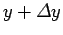, whereis a relatively small division. Let us call this number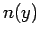. Now, the number of photons which strike a region of the film in a given time interval is equivalent to the intensity of the light illuminating that region of the film multiplied by the area of the region, since each photon carries a fixed amount of energy. Hence, in order to reconcile the classical and quantum viewpoints, we need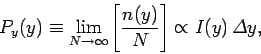(69)

where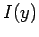is given in Eq. (67). Here,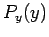is the probability that a given photon strikes the film betweenand. This probability is simply a number between 0 and 1. A probability of 0 means that there is no chance of a photon striking the film betweenand, whereas a probability of 1 means that every photon is certain to strike the film in this interval. Note that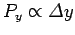. In other words, the probability of a photon striking a region of the film of widthis directly proportional to this width. Actually, this is only true as long asis relatively small. It is convenient to define a quantity known as the probability density,, which is such that the probability of a photon striking a region of the film of infinitesimal widthis. Now, Eq. (69) yields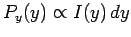, which gives. However, according to Eq. (66),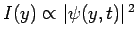. Thus, we obtain(70)

In other words, the probability density of a photon striking a given point on the film is proportional to the modulus squared of the wavefunction at that point. Another way of saying this is that the probability of a measurement of the photon's distance from the centerline, at the location of the film, yielding a result betweenand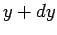is proportional to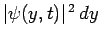.

Note that, in the quantum mechanical picture, we can only predict the probability that a given photon strikes a given point on the film. If photons behaved classically then we could, in principle, solve their equations of motion and predict exactly where each photon was going to strike the film, given its initial position and velocity. This loss of determinancy in quantum mechanics is a direct consequence of wave-particle duality. In other words, we can only reconcile the wave-like and particle-like properties of light in a statistical sense. It is impossible to reconcile them on the individual particle level.

In principle, each photon which passes through our apparatus is equally likely to pass through one of the two slits. So, can we determine which slit a given photon passed through? Well, suppose that our original interference experiment involves sending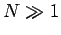photons through our apparatus. We know that we get an interference pattern in this experiment. Suppose that we perform a modified interference experiment in which we close off one slit, sendphotons through the apparatus, and then open the slit and close off the other slit, and sendphotons through the apparatus. In this second experiment, which is virtually identical to the first on the individual photon level, we know exactly which slit each photon passed through. However, the wave theory of light (which we expect to agree with the quantum theory in the limit) tells us that our modified interference experiment will not result in the formation of an interference pattern. After all, according to wave theory, it is impossible to obtain a two-slit interference pattern from a single slit. Hence, we conclude that any attempt to measure which slit each photon in our two-slit interference experiment passes through results in the destruction of the interference pattern. It follows that, in the quantum mechanical version of the two-slit interference experiment, we must think of each photon as essentially passing through both slits simultaneously.Next: Classical Particles Up: Wave-Particle Duality Previous: Classical Interference of Light
Richard Fitzpatrick 2010-07-20